• This is an assessment test.
• These tests focus on geometry and mensuration and are meant to indicate your preparation level for the subject.
• Kindly take the tests in this series with a pre-defined schedule.

## Geometry and Mensuration: Test 11

Congratulations - you have completed Geometry and Mensuration: Test 11. You scored %%SCORE%% out of %%TOTAL%%. You correct answer percentage: %%PERCENTAGE%% . Your performance has been rated as %%RATING%%
 Question 1
ABC is a right angled triangle, right angled at C and p is the length of the perpendicular from C on AB. If a, b and c are the lengths of the sides BC, CA and AB respectively, then
 A $\displaystyle \frac{1}{{{p}^{2}}}=\frac{1}{{{b}^{2}}}-\frac{1}{{{a}^{2}}}$ B $\displaystyle \frac{1}{{{p}^{2}}}=\frac{1}{{{a}^{2}}}+\frac{1}{{{b}^{2}}}$ C $\displaystyle \frac{1}{{{p}^{2}}}+\frac{1}{{{a}^{2}}}=\frac{1}{{{b}^{2}}}$ D $\displaystyle \frac{1}{{{p}^{2}}}=\frac{1}{{{a}^{2}}}-\frac{1}{{{b}^{2}}}$
Question 1 Explanation: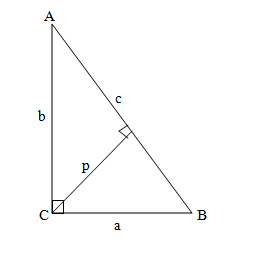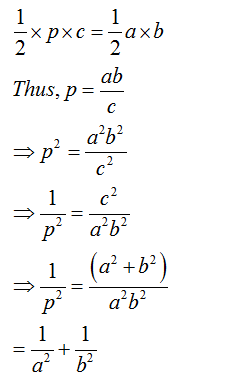Question 2
$\displaystyle \begin{array}{l}If\vartriangle ABC\text{ }is\text{ }similar\text{ }to\vartriangle DEF\text{ }such\text{ }that\text{ }BC=\text{ }3\text{ }cm,\text{ }\\EF=\text{ }4cm\text{ }and\text{ }area\text{ }of\vartriangle ABC=\text{ }54\text{ }c{{m}^{2}},\text{ }\\then\text{ }the\text{ }area\text{ }of\vartriangle DEF\text{ }is:\end{array}$
 A 66 cm2 B 78 cm2 C 96 cm2 D 54 cm2
Question 2 Explanation:
In case of similar triangles, the ratio of the areas of the triangles is equal to the square of the sides in proportion.
The ratio of the area of ΔABC  to ΔDEF = 32: 42 =  9:16
The Area of ΔDEF= (16/9)x54 = 96 cm2 Thus, the correct option (c)
 Question 3
$\displaystyle \begin{array}{l}In\,ABC,\angle A=\text{ }{{90}^{o}}and\,AD\bot BC\,where\text{ }D\text{ }lies\text{ }on\text{ }BC.\text{ }\\If\text{ }BC=\text{ }8\text{ }cm,\text{ }AC=\text{ }6cm,\text{ }\\then\,\vartriangle ABC:\,\vartriangle ACD=\text{ }?\end{array}$
 A 4: 3 B 25: 16 C 16: 9 D 25: 9
Question 3 Explanation: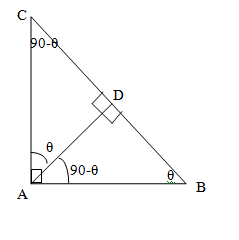From the figure the ΔABC and ΔACD are similar triangles by AAA similarity.
In case of similar triangles, the ratio of the areas of the triangles is equal to the square of the sides in proportion.
We have ΔABC/ΔACD =82/62= 16/9
 Question 4
The areas of two similar triangles ABC and DEF are 20 cm2 and 45 cm2 respectively. If AB= 5cm, then DE is equal to:
 A 6.5 cm B 7.5 cm C 8.5 cm D 5.5 cm
Question 4 Explanation:
In case of similar triangles, the ratio of the areas of the triangles is equal to the square of the sides in proportion.
Hence we have AB2 /DE2 = 20/45 = 4/9
AB/DE = 2/3
Now if AB = 5 cm, then DE = 5 x 3/2 = 7.5cm
 Question 5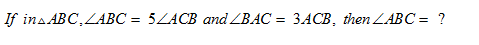A 130o B 80o C 100o D 120o
Question 5 Explanation: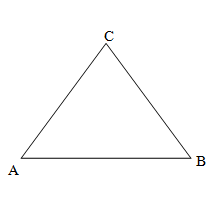We have ∠ABC + ∠BAC + ∠ACB = 1800
=> 5∠ ACB + 3∠ACB + ∠ ACB = 1800
=> 9 ∠ ACB = 1800
=>∠ ACB = 200
So ∠ ABC = 5 ∠ ACB = 5 × 20 = 1000.
Once you are finished, click the button below. Any items you have not completed will be marked incorrect.
There are 5 questions to complete.
 ← List →
 1 2 3 4 5 End

### Want to explore more Arithmetic Tests?

Get Posts Like This Sent to your Email
Updates for Free Live sessions and offers are sent on mail. Don't worry: we do not send too many emails..:)
Get Posts Like This Sent to your Email
Updates for Free Live sessions and offers are sent on mail. Don't worry: we do not send too many emails..:)